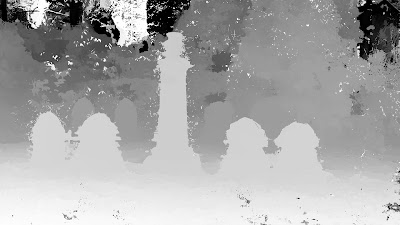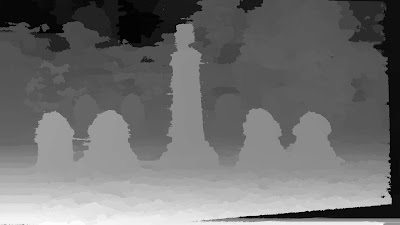## Thursday, April 14, 2016

### Multi View Stereo (MVS) vs Two View Stereo

In this post, we are gonna compare Structure from Motion 10 (SfM10) + Depth Map Automatic Generator 8b (DMAG8b) [Multi View Stereo] against Epipolar Rectification 9b (ER9b) + Depth Map Automatic Generator 5 (DMAG5) [Two View Stereo].

DMAG8b creates a depth map from a series of frames using the nvm file that SfM10 produces. DMAG5 creates a depth map from an image pair rectified by ER9b. It should be noted that DMAG8b is basically the multi view version of DMAG5 (they are using the same parameters).

One would think that the combo SfM10+DMAG8b would create a more accurate depth map because there are more images involved but it is not a given.

Let's first see what kind of depth map the combo SfM10+DMAG8b produces:3D reconstruction by SfM10 using nbr of trials (AC-RANSAC) = 10000, max number of iterations (Bundle Adjustment) = 1000, min separation angle = 1.5, and max reprojection error = 16.Depth map obtained by DMAG8b using near plane = 0, far plane = 0, number of planes = 0, radius = 36, alpha = 0.9, truncation (color)= 30, truncation (gradient) = 10, and epsilon = 4.

I wouldn't worry too much about the wrong depths way back in the background as the actual far plane is probably deeper than the calculated far plane (from the 3D reconstruction).

Now, let's look at the depth map produced by the combo ER9b+DMAG5:

The initial pair chosen by SfM10 is the stereo pair we are gonna use in ER9b. In order to get a depth map (with DMAG5) that shows the foreground as white and the background as black, we need to figure out which one is the left image and which one is the right image. That's pretty easy to tell just by looking at the images.

The min and max disparity that ER9b outputs can be plugged in into DMAG5 as is. If they don't make sense (the range is way too large compared to reality), it definitely means that wrong matches were kept as inliers (the rectified images should be ok though). In that case, one can either try to use ER9 instead of ER9b (don't forget to negate and switch the min and max disparities since ER9 uses a different convention than ER9b for disparities) or use DF2 to manually compute the min and max disparities. In our case, ER9b gives min disparity = -8 and max disparity = 139, which looks mighty fine. Note that the initial pair chosen by SfM10 was the pair of images with index 2 and 3, which means that image 2 is the reference image. For ER9b and DMAG5, the left image is the one with index 3 and the right image the one with index 2. No big deal if you switch those up as the only thing that's gonna happen is that you're gonna get an inverted depth map where black is foreground and white is background.Disparity map obtained by DMAG5 using radius = 36, alpha = 0.9, truncation (color)= 30, truncation (gradient) = 10, and epsilon = 4.

This depth map was obtained using the exact same parameters (in DMAG5) as the previous one (DMAG8b). The only difference really is that here (DMAG5) the left and right depth maps are computed in order to detect occlusions which are then erased and filled in using neighboring depths (inpainting).

Which depth map is best? Well, I will let you decide but one can certainly say that the depth map produced by the combo SfM10+DMAG8b is not really better than the depth map produced by the combo ER9b+DMAG5. It should also be noted that, in general, the combo SfM10+DMAG8b is much slower than the combo ER9b+DMAG5.